# Z.TEST Function

Calculates the one-tailed P-value of a Z-test

## What is the Z Test Excel Formula?

The Z TEST Excel formula is categorized under Excel Statistical functions. It will calculate the one-tailed P-value of a Z-test.

As a financial analyst, the Z.TEST function is useful for various analyses. For example, we can decide if we should invest in a particular stock when it provides a specific average daily return.

### Z Test Formula

=Z.TEST(array,x,[sigma])

The Z.TEST function uses the following arguments:

1. Array (required argument) – This is the array or range of data against which we need to test x. The array is a set of values against which the hypothesized sample mean is to be tested.
2. X (optional argument) – This is the hypothesized sample. It is the value to be tested.
3. Sigma (optional argument) – This represents the population’s standard deviation if this is known. If omitted, the function uses the sample standard deviation.

### How to use the Z.TEST Function in Excel?

To understand the uses of the Z.TEST function, let’s consider an example:

#### Example – Z Test Excel Formula

Suppose we are given the following data: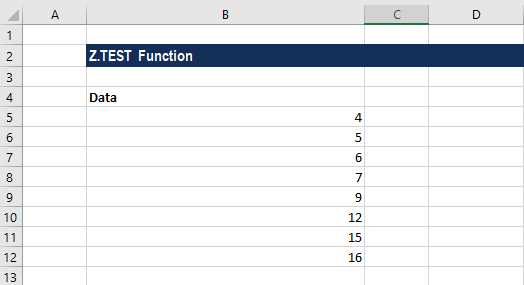To calculate the one-tailed probability-value of a z-test for the data with a hypothesized population mean of 4, we will use the following formula: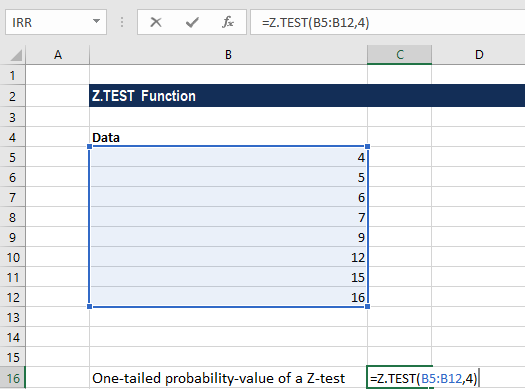We get the result below: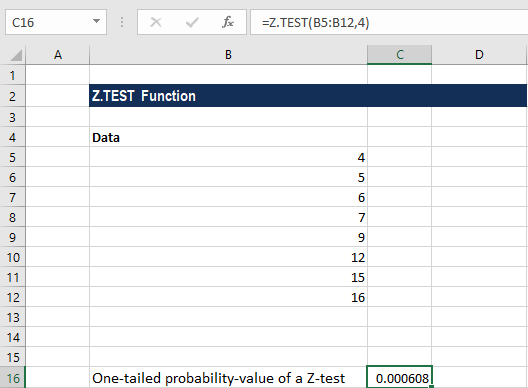For calculating the two-tailed probability-value of a z test Excel for the above data with a hypothesized population mean of 4, we will use the following formula: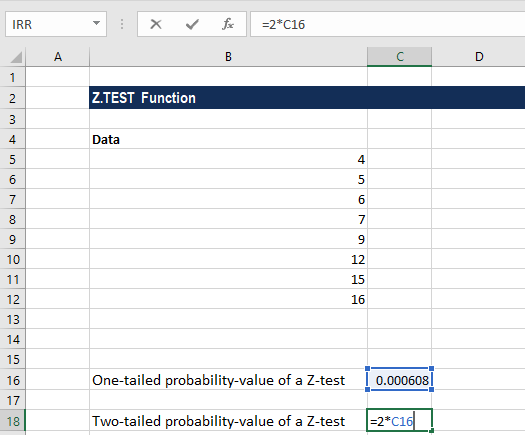We get the result below: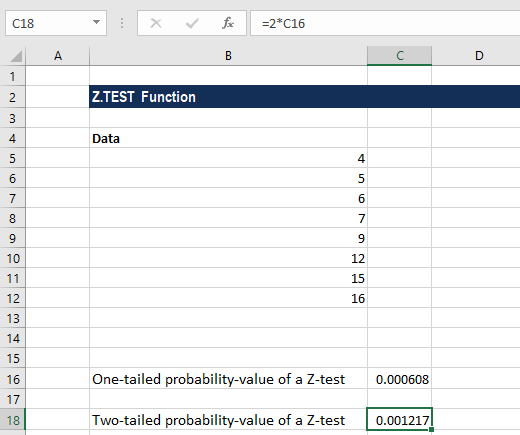### Things to remember about the Z TEST Excel Function

1. Z.TEST represents the probability that the sample mean would be higher than the observed value AVERAGE(array), when the underlying population mean is μ0. From the symmetry of the normal distribution, if AVERAGE(array) < x, Z.TEST will return a value greater than 0.5.
2. #VALUE! error – Occurs when the value of x or the value of sigma is non-numeric.
3. #NUM! error – This error occurs when the sigma argument provided is equal to zero.
4. #N/A error – Occurs when the provided array is empty.
5. #DIV/0! error – Occurs when:
• The sigma is not provided and the standard deviation of the supplied array is zero.
• The given array contains just one value.

### Free Excel Course

Work your way toward becoming an expert financial analyst by checking our Free Excel Crash Course. Learn how to use Excel functions and create sophisticated financial analysis and financial modeling.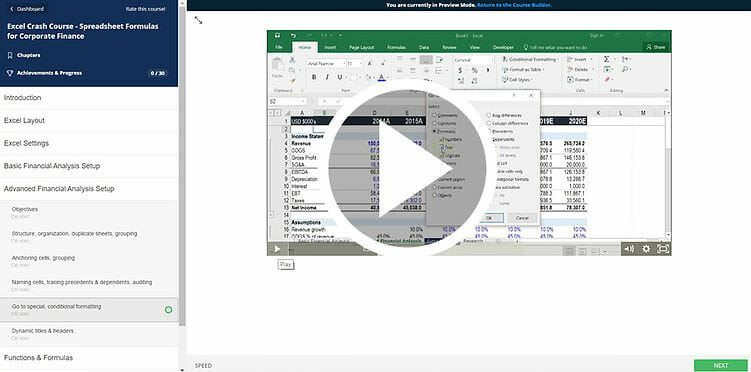Thanks for reading CFI’s guide to the Z Test Excel formula! By taking the time to learn and master these functions, you’ll significantly speed up your financial analysis. To learn more, check out these additional CFI resources:

• Excel Functions for Finance